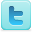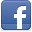﻿ Georg Simon Ohm: The Discovery of Ohm's Law

Georg Simon Ohm
The Discovery of Ohm's Law
Ohm's Law Experiments

### Georg Ohm's DiscoveriesOhm's Law Triangle Helps remember Ohm’s law Black-slon - CC 4.0
Georg Ohm, German mathematician and physicist, began his important publications in 1825.

In his first paper published in 1825, Ohm examines the decrease in the electromagnetic force produced by a wire as the length of the wire increased. The paper deduced mathematical relationships based purely on the experimental evidence that Ohm had tabulated.

In two important papers in 1826, Ohm gave a mathematical description of conduction in circuits modelled on Fourier's study of heat conduction. These papers continue Ohm's deduction of results from experimental evidence and, particularly in the second, he was able to propose laws which went a long way to explaining results of others working on galvanic electricity. The second paper certainly is the first step in a comprehensive theory which Ohm was able to give in his famous book published in the following year.

What is now known as Ohm's law appeared in this famous book Die galvanische Kette, mathematisch bearbeitet (1827) in which he gave his complete theory of electricity. The book begins with the mathematical background necessary for an understanding of the rest of the work. We should remark here that such a mathematical background was necessary for even the leading German physicists to understand the work, for the emphasis at this time was on a non-mathematical approach to physics. We should also remark that, despite Ohm's attempts in this introduction, he was not really successful in convincing the older German physicists that the mathematical approach was the right one.

As stated above, this work included “Ohm’s Law” theory: The relationship of a current passing through most materials is directly proportional to the potential difference applied across the material.

Although Ohm's work strongly influenced theory, at first it was received with little enthusiasm. However, his work was eventually recognised by the Royal Society with its award of the Copley Medal in 1841. He became a foreign member of the Royal Society in 1842, and in 1845 he became a full member of the Bavarian Academy.

### Ohm's Law

Ohm's law, named after its discoverer, states that the potential difference V between the ends of a conductor or resistor R and the current I flowing through R are proportional at a given temperature:In other words, where V is the voltage and I is the current; the above equation yields the proportionality constant R, which is the electrical resistance of the device.

The law is strictly true only for resistors whose resistance does not depend on the applied voltage, which are called ohmic or ideal resistors or ohmic devices. Ohm's law is never completely accurate, if R is assumed to be constant, for "real world" devices, because no real device is an ohmic device for every voltage and current - at some level, the device will open or short, for example, by burning up or arcing. Moreover, temperature is an important factor determining the accuracy of Ohm’s law. When the temperature of the metal increases, the collisions between electrons and atoms increase, so that when a substance heats up because of electricity flowing through it (or by whatever heating process), the resistance will increase.

The relation V / I = R even holds also for non-ohmic devices, but then the resistance R depends on V and is no longer a constant. To check whether a given device is ohmic or not, one plots V versus I and compares the graph to a straight line through the origin.

It is important to note that Ohm's law is not an actual mathematically derived law, but an observation supported by significant empirical evidence.

In 1845 Gustav Kirchhoff (1824 - 1887), German physicist, announced the discovery of Kirchhoff's laws, which allow calculation of the currents, voltages, and resistances of electrical networks. Extending the theory of Georg Ohm, he generalized the equations describing current flow to the case of electrical conductors in three dimensions. In further studies he demonstrated that current flows through a conductor at the speed of light.

### Ohm's Law Experiments

Ohm's Law Experiments
Ohm's Law Experiment - MSU Department of Astronomy and Physics
Current/Voltage Characteristics and Ohm's Law - Davidson Physics
Ohm's Law Pie Chart and Calculators - 12volt.com
Ohm's Law - R. Victor Jones
Ohm’s law, electrical power, emf and internal resistance - PMB
Ohm’s Law Relationships - Walter Banzhaf, E.K. Smith, Winfield Young
Ohm's Law - Randall J. Scalise
Ohm's Law - JL Stanbrough
Ohms Law Ohmic Resistors versus Lightbulbs - UBC

Kirchhoff's Laws Experiments
Kirchhoff's Laws - University of Guelph, Department of Physics
Ohm's Law & Kirchhoff's Rules - Chris Odom, Clemson University

Ohm's Law Background
Ohm's Law - National High Magnetic Field Laboratory
Ohm's Law - HyperPhysics
Ohm's Law - University of Guelph, Department of Physics
Ohm's Law Pie Chart and Calculators - 12volt.com
Ohm's Law Demo - Science Joy Wagon

Georg Ohm Biographies
Georg Simon Ohm - MacTutor

### Books